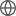Old Web
English
We present the KMOS Galaxy Evolution Survey (KGES), a $K$-band Multi-Object Spectrograph (KMOS) study of the H$\alpha$ and [NII] emission from 288 $K$ band-selected galaxies at $1.2 \lesssim z \lesssim 1.8$, with stellar masses in the range $\log_{10}(M_{*}/\rm{M}_{\odot})\approx$9-11.5. In this paper, we describe the survey design, present the sample, and discuss the key properties of the KGES galaxies. We combine KGES with appropriately matched samples at lower redshifts from the KMOS Redshift One Spectroscopic Survey (KROSS) and the SAMI Galaxy Survey. Accounting for the effects of sample selection, data quality, and analysis techniques between surveys, we examine the kinematic characteristics and angular momentum content of star-forming galaxies at $z\approx1.5$, $\approx1$ and $\approx0$. We find that stellar mass, rather than redshift, most strongly correlates with the disc fraction amongst star-forming galaxies at $z \lesssim 1.5$, observing only a modest increase in the prevalence of discs between $z\approx1.5$ and $z\approx0.04$ at fixed stellar mass. Furthermore, typical star-forming galaxies follow the same median relation between specific angular momentum and stellar mass, regardless of their redshift, with the normalisation of the relation depending more strongly on how disc-like a galaxy's kinematics are. This suggests that massive star-forming discs form in a very similar manner across the $\approx$ 10 Gyr encompassed by our study and that the inferred link between the angular momentum of galaxies and their haloes does not change significantly across the stellar mass and redshift ranges probed in this work.